# Trigonometry Formulas for Functions, Ratios and Identities PDF

Trigonometry is a branch of mathematics that deal with angles, lengths and heights of triangles and relations between different parts of circles and other geometrical figures. Maths Formulas – Trigonometric Ratios and identities are very useful and learning the below formulae help in solving the problems better. Trigonometry formulas are essential for solving questions in Trigonometry Ratios and Identities in Competitive Exams.

Trigonometric Identities are equalities that involve trigonometric functions and are true for every value of the occurring variables where both sides of the equality are defined. Geometrically, these are identities involving certain functions of one or more angles.

Trigonometric Ratio relationship between the measurement of the angles and the length of the side of the right triangle. These formulas relate lengths and areas of particular circles or triangles. On the next page you’ll find identities. The identities don’t refer to particular geometric figures but hold for all angles.

## Trigonometry Formulas

#### Formulas for arcs and sectors of circles

You can easily find both the length of an arc and the area of a sector for an angle θ in a circle of radius r.

Length of an arc. The length of the arc is just the radius r times the angle θ where the angle is measured in radians. To convert from degrees to radians, multiply the number of degrees by π/180.

Arc = rθ.### Trigonometric Formulas – Right Angle

The most important formulas for trigonometry are those for a right triangle. If θ is one of the acute angles in a triangle, then the sine of theta is the ratio of the opposite side to the hypotenuse, the cosine is the ratio of the adjacent side to the hypotenuse, and the tangent is the ratio of the opposite side to the adjacent side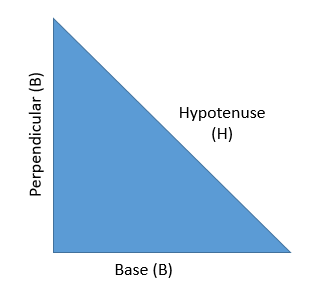Pythagorean theorem, the well-known geometric theorem that the sum of the squares on the legs of a right triangle is equal to the square on the hypotenuse (the side opposite the right angle)—or, in familiar algebraic notation, (P)2 + (B)2 = (H)2

Applying Pythagoras theorem for the given right-angled theorem, we have:

(Perpendicular)2 + (Base)2 = (Hypotenuse)2

(P)2 + (B)2 = (H)2

The trigonometric properties are given belowMagical Hexagon for Trigonometry Identities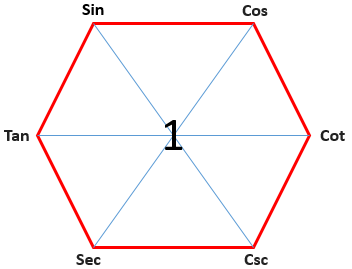Clock Wise: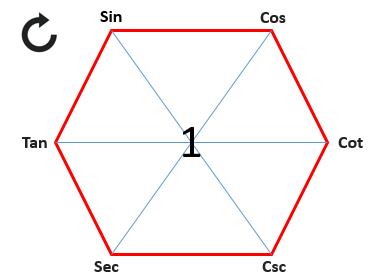• tan(x) = sin(x) / cos(x)
• sin(x) = cos(x) / cot(x)
• cos(x) = cot(x) / csc(x)
• cot(x) = csc(x) / sec(x)
• csc(x) = sec(x) / tan(x)
• sec(x) = tan(x) / sin(x)

Counterclock Wise: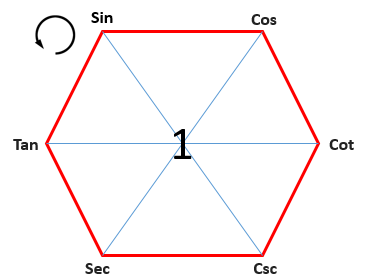• cos(x) = sin(x) / tan(x)
• sin(x) = tan(x) / sec(x)
• tan(x) = sec(x) / csc(x)
• sec(x) = csc(x) / cot(x)
• csc(x) = cot(x) / cos(x)
• cot(x) = cos(x) / sin(x)

###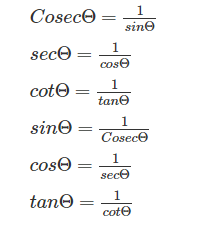### Square Law FormulasAlong with the knowledge that the two acute angles are complementary, that is to say, they add to 90°, you can solve any right triangle:

• If you know two of the three sides, you can find the third side and both acute angles.
• If you know one acute angle and one of the three sides, you can find the other acute angle and the other two sides.

### Signs of Trigonometric Ratios

A lot of trigonometry formulas are based on the signs of trigonometric ratios, based on the quadrants they lie in. Therefore it becomes extremely essential for us to understand how trigonometric ratios get the positive or negative
sign. The sign is based on the quadrant in which the angle lies.Let us assume an angle of θ1 lying in the 1st quadrant and an angle θ in quadrant one and two combined.
So let us see how signs change with respect to the quadrant they lie in.

• In Q1 all Trigonometric Ratios are positive. (Angles between 00 – 900)
• In Q2 all trigonometric ratios of sinθ and cosecθ are positive. (Angles between 900 – 1800)
• In Q3 all trigonometric ratios of cosθ and secθ are positive. (Angles between 1800 – 2700)
• In Q4 all trigonometric ratios of tanθ and cotθ are positive. (Angles between 2700 – 3600)

θ is the angle made between the x-axis and the line, in the anti-clockwise direction. If we move in the clockwise direction, the angle will be taken as – θ. We know that in quadrant 4, only cosθ and secθ will be positive, the others will be negative, therefore-

• Sin (– θ) = – Sin θ
• Cos (– θ) =  Cos θ
• Tan (– θ) =– Tan θ
• Sec (– θ) = + Sec θ
• Cot (– θ) = – Cot θ

We need to understand that trigonometric ratios would change for angles- 90o ± θ and 270o ± θ and they will remain same for 180o ± θ and 360o ± θ. Let’s see what happens when we add or subtract θ from 90o ± θ and 270o ± θ• Sec (90o + θ ) = Cos θ
• Cot (90o – θ ) = Cos θ
• Tan (90o + θ ) = – Cot  θ
• Tan (90o – θ ) = Cot  θ
• Sec (90o + θ ) = Cosec θ
• Sec (90o + θ ) = Cosec θ
• Sin (270o – θ ) = – Cos θ
• Sin (270o – θ ) = – Cos θ

This is because any angle that is 2700+θ will fall in quadrant 4 and in this quadrant only trigonometric ratios of cos
and sec are positive. So the above will be negative. 2700-θ will fall in the quadrant 3 and in this quadrant trigonometric ratios of tan and cot are positive, so it will again be negative. For 180o ± θ and for 360o ± θ, the signs will remain the same.

• Sin (360o + θ ) = Sin θ
• Sin (360o – θ ) = – Sin θ

For 3600+θ, the angle will complete one full rotation and then lie in quadrant 1 where all trigonometric ratios are positive. So there are 2 important things to remember-

• The sign of the trigonometric ratios change based on the value of θ.
• sin becomes cos and cos becomes sin for 900+θ and for 2700+θ and it remains the same for 1800+θ
and for 3600+θ.

### Trigonometry Formulas | Trigonometric Identities

After looking at the trigonometric ratios, let us move on to trigonometric identities, which are the basics of most trigonometry formulas. The above identities hold true for any value of θ.

Product Identities: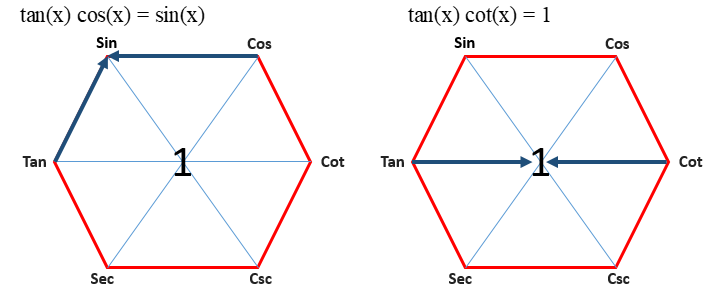### Trigonometry Formulas | Sum and Difference of Angles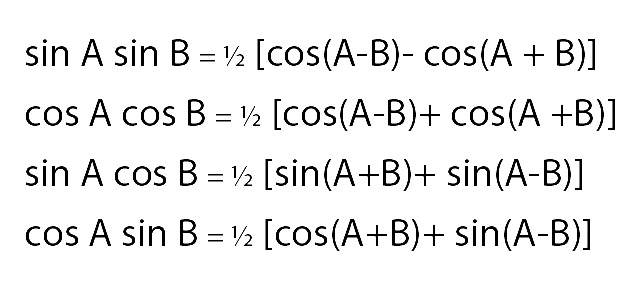### Trigonometry Formulas | Triple Angle Formulas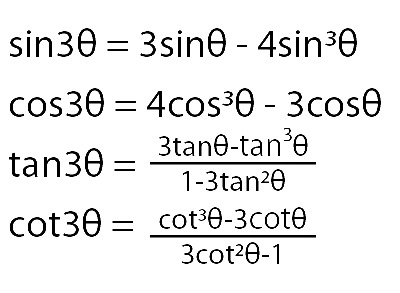### Trigonometry Formulas | Converting Product into Sum and Difference###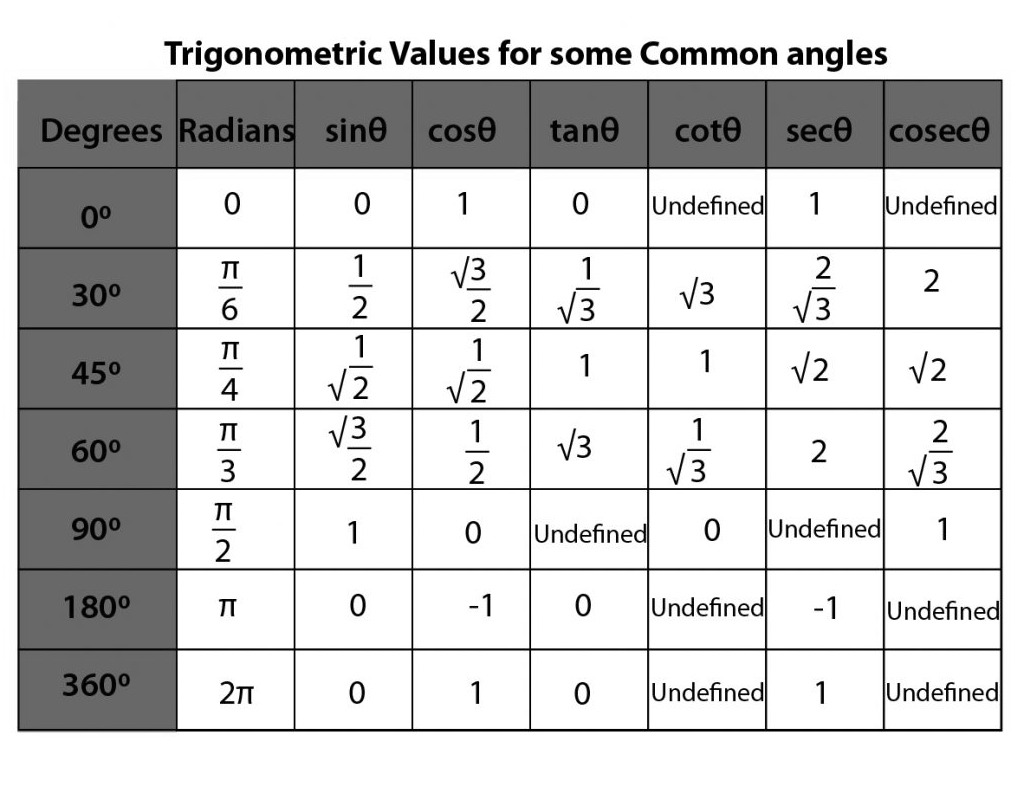Summary of Trigonometric Identities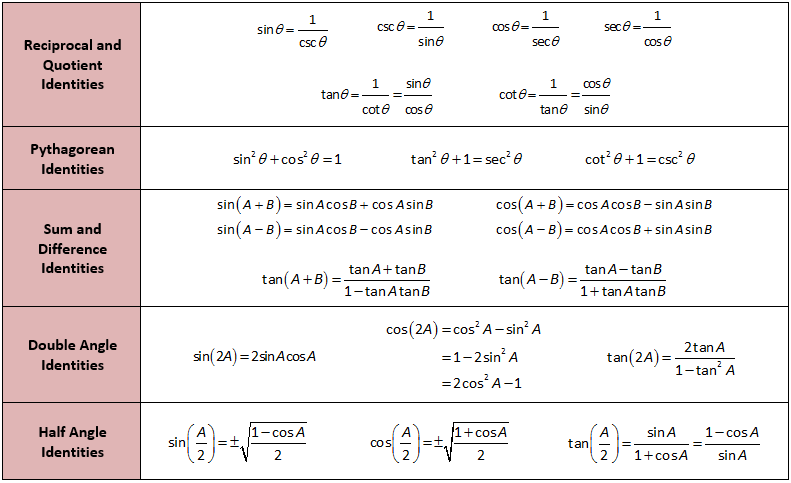### Periodicity and Periodic Identities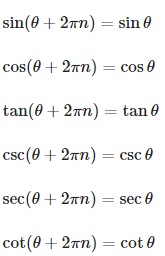### Half angle identities### Complex relations### Inverse trigonometric functions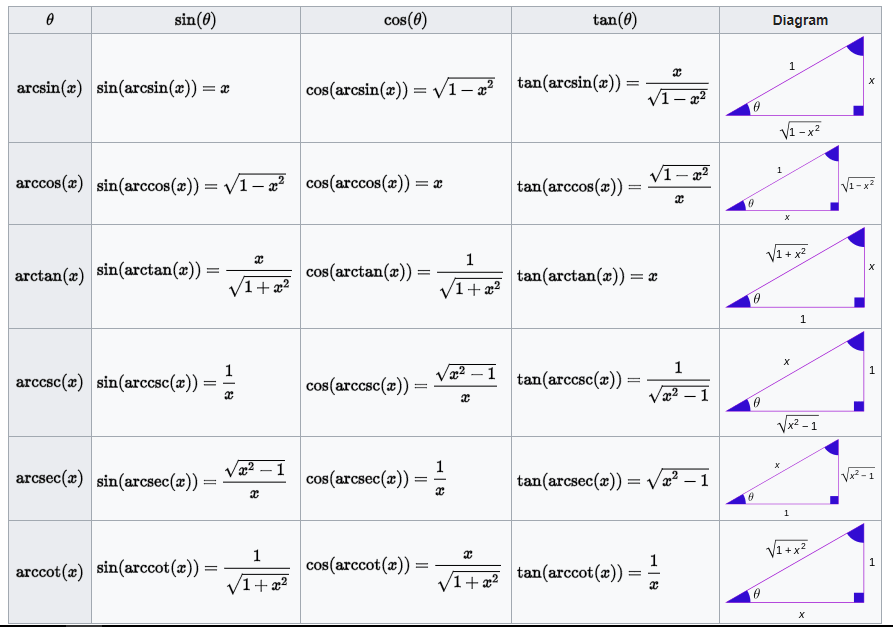### Complimentary angle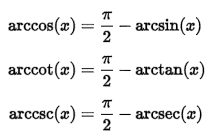### Negative arguments### Reciprocal arguments### Values of Trigonometric Functions